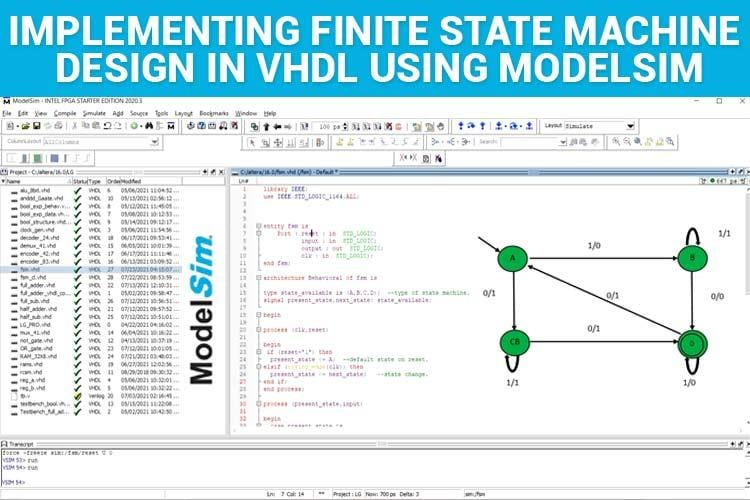# FSMImplementing Finite State Machine Design in VHDL using ModelSimFSM (Finite State Machines) is a simple state machine or a mathematical model of computation.  Each FSM has a finite number of states, inputs,…